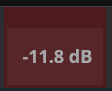# Function to convert MIDI Gain Value to dB for Controller Bar display

I would like to display a dB value in the Controller Bar based on incoming 7-bit MIDI values. I’ve tried to glean how this might be done from the Function reference (available here), but can’t figure it out …

I could get by with seeing 0 … 96 … 127 on my Controller Bar display, but seeing “-∞” or “0dB” or “+7.3dB” would be nicer.

I assume that there is some gain curve being applied, but I’m not so clear on how these work …

Hi Clint,

I looked at this and it is do-able but you must use maths to achieve it and some formatting to keep the result small because log calculations can produce large numbers of decimal places. In the Control Curves there are formulas that you can adapt to use for this. The equation I am referring to is this one

`pow(10, (log(x, 10) * slope - (log(zeroDbPos, 10) * slope)) / 20)`

taken from the “New” curve file. It produces a scalar output, meaning 0.000 to 1.000 would be the output range. This could be used to build an expression but require another conversion using the “to dB(x)” built in function so to simplify I stripped the equation down.

The relevant part of the equation required is

`(log(x, 10) * slope - (log(zeroDbPos, 10) * slope)`

where x = cc(number,channel)

and where zeroDbPos = 96 and slope = 60 , note these values are also shown in the control curve file as declared constants.

filling in the equation for a standard CC number value looks like this (notice the section cc(7,2) represents cc 7 on channel 2).

`log(cc(7,2),10)*60 - (log(96,10) * 60`

This also needs formatting because it makes long number outputs so when you make the string statement you add a formatting element to the script like this where the part of the expression “N1” forces to a single decimal place.

`\$(formatNumber(log(cc(7,2),10)*60 - (log(96,10) * 60),"N1"))`

Then to dress it up you can add the prefix and suffix parts using conditional expressions.

`\$(cc(7,2) > 96 ? "+": " ")\$(formatNumber(log(cc(7,2),10)*60 - (log(96,10) * 60),"N1")) \$(cc(7,2) > 0 ? "dB" : " " )`

The prefix adds the + sign when you exceed 0 db and the suffix adds the “dB” except when at value ∝.

Here it is shown on a Controller Bar button.I think this ought to do it for your setup or anyone who wants to display this type of info. Let me know if it works for you.Dave

4 Likes

Wow.

I would never have gotten there …

Thanks (again) so much!

Genius at work, next Nobel Prize in Mathematics…

1 Like

It works for me, but … I had to make an essential, important, exclusive change: I changed the color of the button …Thanks you, Nobel Dave

1 Like

Here’s my updated controller bar:

I’ve modified some things in the @dave_dore version:

• Didn’t really need the “dB” at the end of each value.

• The regular “dash” character is much shorter than it should be for rendering numbers. I have used a Figure Dash character (“‒” Unicode U+2012) and stripped the “-” character from the number with the abs() function.

• I have shortened displays of less than ‒100 dB by removing the tenths. (“N0” vs “N1”)

• I display 0 dB as +0.0 for consistency (see Mic 1). This keeps the displayed numbers from “jumping around” when turning knobs.

Some details of the title of the left-most block/button:

• The “100%” shows the battery state

• “Chg” says “Charging” (i.e. plugged in to the Mains) as opposed to “BAT” - on battery power.

• “48k” is the sample rate

• The red squares indicated a MUTED track. Note that Mic2 and BkgC are muted.

The actual formula I am using is:

``````\$(cc(0,16) < 1 ? "🟥\n" : "")\$(cc(1,16) >= 96 ? "+" : "‒")
\$(formatNumber(abs(log(cc(1,16),10) * 60 - (log(96,10) * 60)),
cc(1,16) < 3 ? "N0" : "N1"))
``````

… where cc(0,16) is the Enabled state of the route (i.e. Mute) and cc(1,16) is the gain value.

Please note that I have broken the formula above onto three lines for readability. They are entered on a single line in Cantabile.

Also note that the Unicode code point for the red box is Un+1F7E5.

3 Likes

Nice work Clint!!

I see how it would be ultra helpful to have that much feedback displayed on just the controller bar and since I’m used to the sliders being in dB I can visualize where I am on the slider from the dB number displayed very quickly. Thanks for sharing your mods, I certainly learn a lot from other peoples ideas and ways of doing things.Dave

2 Likes